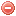# Definition

Net radiation is an input variable of the potential evapotranspiration equation formulated by Penman (1948).

"Net radiation is the difference between incoming and outgoing radiation of both short and long wavelengths. It is the balance between the energy absorbed, reflected and emitted by the earth's surface or the difference between the incoming net shortwave and the net outgoing longwave radiation. Net radiation is normally positive during the daytime and negative during the nighttime. The total daily value for net radiation is almost always positive over a period of 24 hours, except in extreme conditions at high latitudes" (Allen et al. 1998).

# Formula

The calculation of the net radiation requires the following steps (cf. Allen et al. 1998).

The daily extraterrestrial radiation $$R_a=$$ [MJ·m-2·d-1] has to be calculated as follows (Allen et al. 1998):

$R_a=\dfrac{24\cdot{60}}{\pi}\cdot{G_{sc}}\cdot{d_r}\cdot[\omega_s\cdot\sin\varphi\cdot\sin\delta+cos\varphi\cdot\cos\delta\cdot\sin\omega_s]$

where $$G_{sc}$$ is the solar constant, which is equal to 0.082 [MJ·m-2·min-1], $$d_r$$ the inverse relative distance Earth-Sun, $$\omega_s$$ the sunset hour angle [rad], $$\varphi$$ the latitude [rad] , and $$\delta$$ the solar declination [rad].

The inverse relative distance Earth-Sun $$d_r$$ is calculated as follows:

$d_r=1+0.033\cdot\cos\bigg(\dfrac{2\pi}{365}\cdot J\bigg)$

where $$J$$ is the number of the day in the year between 1 (1 January) and 365 or 366 (31 December).

The sunset hour angle $$\omega_s$$ [rad] is calculated as follows:

$\omega_s=\arccos [-\tan\varphi\cdot\tan\delta]$

and the solar declination [rad] $$\delta$$ as follows:

$\delta=0.409\cdot\sin\bigg(\dfrac{2\pi}{365}\cdot J-1.39\bigg)$

The conversion from decimal degrees to radians is obtained as follows:

$\mbox{[Radians]}=\dfrac{\pi}{180} \mbox{ [decimal degrees]}$

The solar radiation $$R_s$$ [MJ·m-2·d-1] can be calculated based on the Angstrom formula (Allen et al. 1998):

$R_s=\bigg(a_s+b_s\cdot\dfrac{n}{N}\bigg)\cdot{R_a}$

where $$n$$ is the actual duration of sunshine [hours], $$N$$ the daylight hours [hours], and $$R_a$$ the extraterrestrial radiation [MJ·m-2·d-1]. $$a_s$$ is a regression constant expressing the fraction of extraterrestrial radiation reaching the earth on an overcast day $$(n=0)$$. $$a_s+b_s$$ is the fraction of extraterrestrial radiation reaching the earth on a clear day $$(n=N)$$. When no actual solar radiation data are available, the values $$a_s=0.25$$ and $$b_s=0.50$$ are recommended (Allen et al. 1998).

Solar radiation $$R_s$$ can also be derived from air temperature differences as follows (Allen et al. 1998):

$R_s=R_a\cdot{k_{Rs}\cdot\sqrt{T_{max}-T_{min}}}$

where $$R_a$$ is the extraterrestrial radiation [MJ·m-2·d-1], $$k_Rs$$ an adjustment coefficient [°C^-0.5] which is usually comprised between 0.16 ("interior" locations) and 0.19 ("coastal" locations), $$T_{max}$$ maximum air temperature [°C] and $$T_{min}$$ minimum air temperature [°C].

The clear-sky solar radiation $$R_{so}$$ [MJ·m-2·d-1] can be calculated as follows when $$a_s$$ and $$b_s$$ values (cf.solar radiation?) are not available (Allen et al. 1998):

$R_{so}=(0.75+2\cdot{10^{-5}}\cdot{z})\cdot{R_a}$

where $$z$$ is elevation above sea level [m].

The net shortwave radiation $$R_{ns}$$ [MJ·m-2·d-1] is calculated as follows (Allen et al. 1998):

$R_{ns}=(1-\alpha)\cdot{R_s}$

where $$\alpha$$ is the albedo or canopoy reflection coefficient, which is usally between 0.20 and 0.25 for green vegetation covers, and $$R_{s}$$ the incoming solar radiation? [MJ·m-2·d-1].

The net longwave radiation $$R_{nl}$$ [MJ·m-2·d-1] is calculated as follows (Allen et al. 1998):

$R_{nl}=\sigma\cdot\bigg(\dfrac{T_{max}^4+T_{min}^4}{2}\bigg)\cdot\Big(0.34-0.14\cdot\sqrt{e_a}\Big)\cdot\bigg(1.35\cdot\dfrac{R_s}{R_{so}}-0.35\bigg)$

where $$\sigma$$ is the Stefan-Bolzmann constant, which is equal to $$4.903\cdot{10^{-9}}$$ [MJ·K-4·m-2·d-1], $$T_{max}$$ and $$T_{min}$$ maximal and minimal temperature [K] during the 24-hr period, $$e_a$$ actual vapor pressure [kPA], $$R_s$$ solar radiation? [MJ·m-2·d-1], and $$R_{so}$$ clear-sky solar radiation? [MJ·m-2·d-1].
$$\dfrac{R_s}{R_{so}}$$ is limited to 1.

Finally, the net radiation $$R_n$$ [MJ·m-2·d-1] can be calculated as follows (Allen et al. 1998):

$R_n=R_{ns}-R_{nl}$

where $$R_{ns}$$ [MJ·m-2·d-1] is net shortwave radiation? and $$R_{nl}$$ [MJ·m-2·d-1] net longwave radiation.

# Reference

Allen et al. (1998)

### Bibliography SearchNo results!

No results were found. Are you sure you searched for a tag?

### Symbols

 Variable Description Unit $$T$$ air temperature °C $$T_{dew}$$ dew point temperature °C $$H$$ air humidity % $$P$$ rainfall mm $$U$$ windspeed m/s $$w$$ days since last rain (or rain above threshold) d $$rr$$ days with consecutive rain d $$\Delta t$$ time increment d $$\Delta{e}$$ vapor pressure deficit kPa $$e_s$$ saturation vapor pressure kPa $$e_a$$ actual vapor pressure kPa $$p_{atm}$$ atmospheric pressure kPa $$PET$$ potential evapotranspiration mm/d $$r$$ soil water reserve mm $$r_s$$ surface water reserve mm $$EMC$$ equilibrium moisture content % $$DF$$ drought factor - $$N$$ daylight hours hr $$D$$ weighted 24-hr average moisture condition hr $$\omega$$ sunset hour angle rad $$\delta$$ solar declination rad $$\varphi$$ latitude rad $$Cc$$ cloud cover Okta $$J$$ day of the year (1..365/366) - $$I$$ heat index - $$R_n$$ net radiation MJ⋅m-2⋅d-1 $$R_a$$ daily extraterrestrial radiation MJ⋅m-2⋅d-1 $$R_s$$ solar radiation MJ⋅m-2⋅d-1 $$R_{so}$$ clear-sky solar radiation MJ⋅m-2⋅d-1 $$R_{ns}$$ net shortwave radiation MJ⋅m-2⋅d-1 $$R_{nl}$$ net longwave radiation MJ⋅m-2⋅d-1 $$\lambda$$ latent heat of vaporization MJ/kg $$z$$ elevation m a.s.l. $$d_r$$ inverse relative distance Earth-Sun - $$\alpha$$ albedo or canopy reflection coefficient - $$\Delta$$ slope of the saturation vapor pressure curve kPa/°C $$Cc$$ cloud cover eights $$ROS$$ rate of spread m/h $$RSF$$ rate of spread factor - $$WF$$ wind factor - $$WRF$$ water reserve factor - $$FH$$ false relative humidity - $$FAF$$ fuel availability factor - $$PC$$ phenological coefficient -

 Suffix Description $$-$$ mean / daily value $$_{max}$$ maximum value $$_{min}$$ minimum value $$_{12}$$ value at 12:00 $$_{13}$$ value at 13:00 $$_{15}$$ value at 15:00 $$_{m}$$ montly value $$_{y}$$ yearly value $$_{f/a}$$ value at fuel-atmosphere interface $$_{dur}$$ duration $$_{soil}$$ value at soil level

 Constant Description $$e$$ Euler's number $$\gamma$$ psychrometric constant $$G_{SC}$$ solar constant $$\sigma$$ Stefan-Bolzmann constant